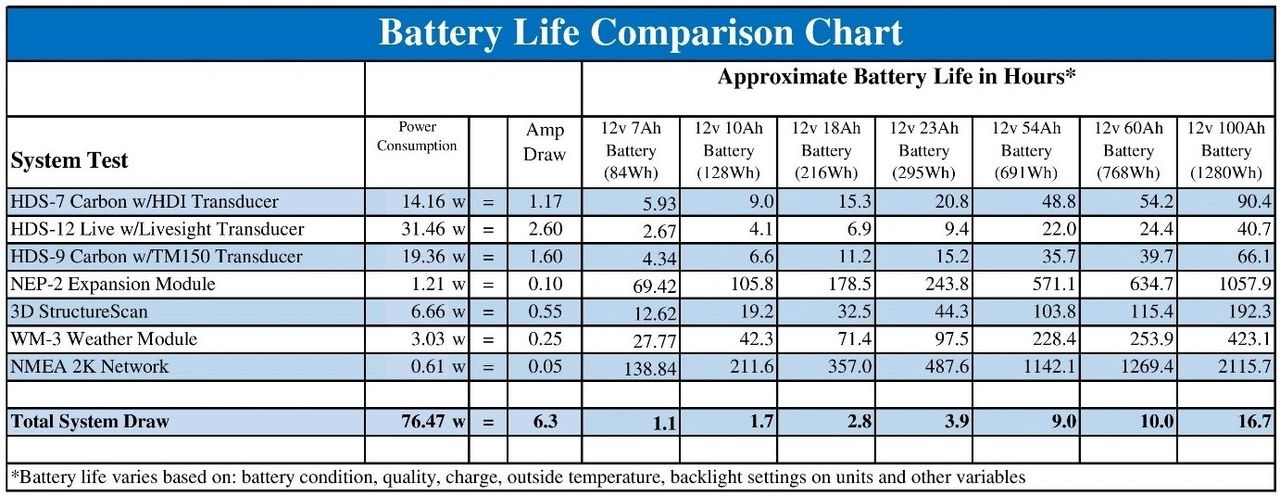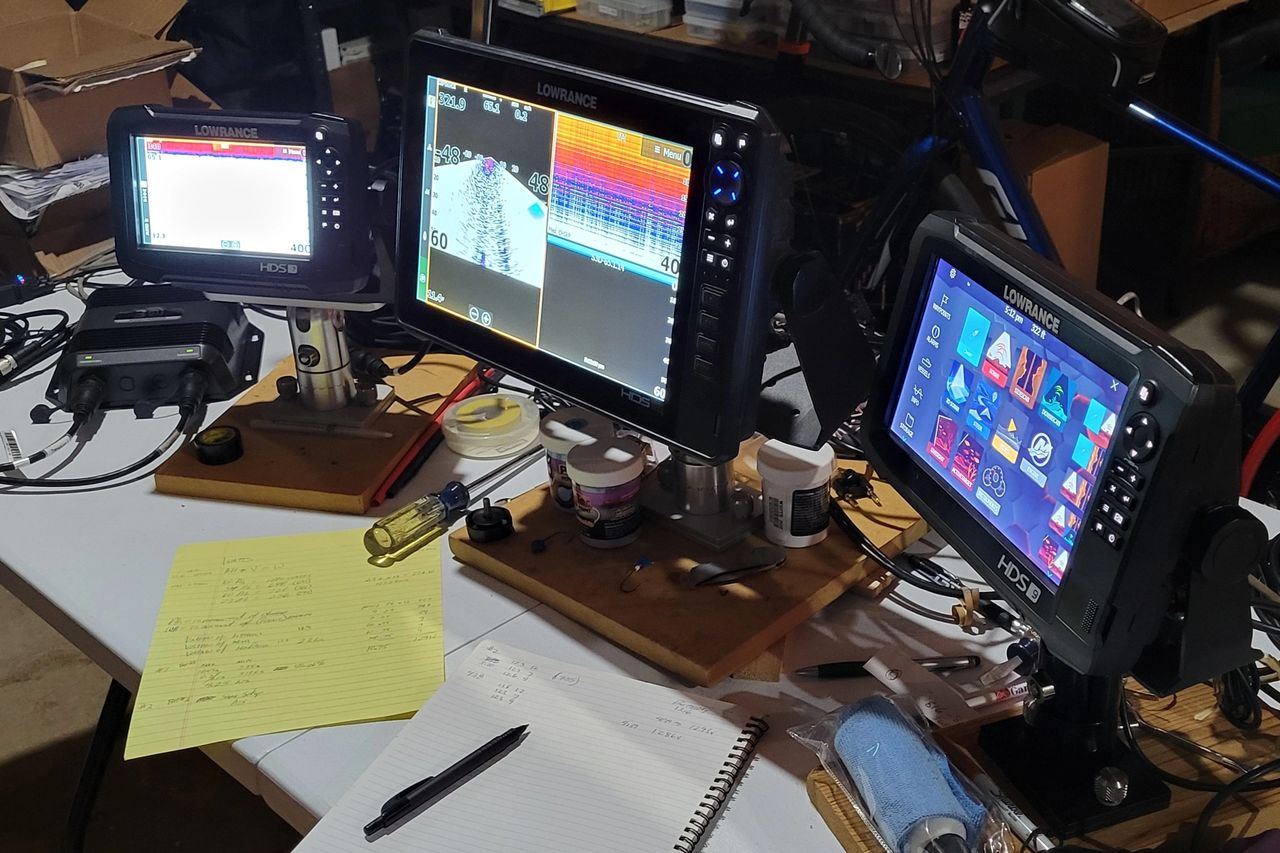# What Size Lithium (LiFePO₄) Battery Do I Really Need on My Boat?

I have spent the last two years validating the use of lithium iron phosphate batteries (LiFePO4) and have learned a great deal and continue to do so when it comes to trying to determine amp hours (Ah) requirements for a given Lowrance system. One of the first things I learned is that the draw of the units will vary as direct result of the power of the battery supply.  It is also important to note that the draw numbers that Lowrance provides under the specifications of each unit are only a reference number at a given voltage supplied and that will vary between each unit based on the given power supplied.

To determine the actual power draw of each product and to do this accurately, I learned that one must measure the power draw from the unit as well as the supplied voltage from the battery at the same time. To do this I simply used two multi-meters and I measured the power draw from the unit and then the power supplied from the battery at the same time.Initial test setup with two multimeters

What I found was with the Dakota Lithium batteries I was using, they started out consistently while supplying a load, right at 13.2v and the test would then conclude when that number would drop to an output of around 10.8v. So rather than creating an equation by taking a number of samplings every time the voltage supply would slightly drop, to make this simpler I just took the average of the range measured (13.2v ~10.8v) and used the average of the measured range for the supplied voltage.

Additionally, I also learned was that as the supply voltage from the battery gradually dropped over time, the amp drawn from the units would increase at the same time to maintain the same current. So once again, rather than creating a complex formula with many variables, I found the best way to come up with a simple way to calculate the amp draw was to also average the results over the length of the test.

So here are some actual results from using a Lowrance HDS-12 Live unit with a HST skimmer plugged into it with GPS running and using a Dakota Lithium 12v7Ah battery for the power source. The Dakota 12v7Ah battery had a resting voltage reading of 13.5v before test, and under load would then settle in the 13.2-13.25v range to start the test:

Supply voltage = 13.2v start, end supply voltage 10.82v

Power draw of HDS-12 Live = 1.58a at start, end draw 1.82a at test completion

In this particular test, the average supply voltage of the battery was 12.1v and the average power draw was 1.7a, so then what does one then do with this data?

I now have the power draw of the particular Lowrance unit in amps, the power supplied is in volts and the rating of the batteries is in amp hours (Ah).  If you look closely at a Dakota Lithium battery, you will also see that they list the output watts as well, which is derived from taking the amp hours (Ah) of the battery and then multiplying that by the rated voltage, which on a Dakota is 12.8v. To handle these different sets of data, I took power draw of the particular unit and then converted that to watts by multiplying that number by the average supplied voltage of the Dakota battery of 12.1 volts.This all worked fine as I first tested this on a single HDS-12 Live with a Dakota 12v7Ah battery, but I also knew that the goal of this exercise was to determine the power draw and battery requirements of an entire system that one would see on a boat. What I then analyzed was a typical or even above normal boat setup which consists of three MFD’s, transducers, hubs, NMEA, etc using a 23Ah Dakota Lithium battery. The draw was measured for each device, combined and then converted to watts. The output wattage of the battery was then divided by the draw of that system in watts and the outcome is then the runtime for that battery. In this test the calculated runtime was 3.9 hours and actual runtime achieved was 4 hours.Test rig setup

From what I am seeing many anglers are turning away from buying lithium (LiFePO4) iron phosphate batteries because they are being told they need to go buy a 100Ah or 125Ah battery, when in actuality a 54Ah or 60 Ah battery may do them just fine.  If I take that same boat setup and connect it to a 100Ah LiFePO4 battery, the runtime will be 16.7 hours, which is much more than what I would expect most anglers would require. That same setup using a 54Ah battery would yield 9.0 hours and a 60 Ah battery would yield 10.0 hours. The bottom-line is don’t just assume you can’t afford the transition to lithium, in many cases a mid-powered battery will serve you just fine.

## Be The First to Know

Get access to exclusive deals, hear about new products before anyone else, and enjoy tips, tricks, and DIY hacks from our community of experts.